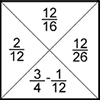Search by Topic

Resources tagged with Equivalent fractions, decimals and percentages similar to The Greedy Algorithm:

Filter by: Content type:
Age range:
Challenge level:

There are 7 results

Broad Topics > Fractions, Decimals, Percentages, Ratio and Proportion > Equivalent fractions, decimals and percentagesThe Greedy Algorithm

Age 11 to 14 Challenge Level:

The Egyptians expressed all fractions as the sum of different unit fractions. The Greedy Algorithm might provide us with an efficient way of doing this.Fractions Jigsaw

Age 11 to 14 Challenge Level:

A jigsaw where pieces only go together if the fractions are equivalent.Sept 03

Age 11 to 14 Challenge Level:

What is the last digit of the number 1 / 5^903 ?Repetitiously

Age 11 to 14 Challenge Level:

The number 2.525252525252.... can be written as a fraction. What is the sum of the denominator and numerator?Round and Round and Round

Age 11 to 14 Challenge Level:

Where will the point stop after it has turned through 30 000 degrees? I took out my calculator and typed 30 000 ÷ 360. How did this help?Tiny Nines

Age 14 to 16 Challenge Level:

Find the decimal equivalents of the fractions one ninth, one ninety ninth, one nine hundred and ninety ninth etc. Explain the pattern you get and generalise.Fractions and Percentages Card Game

Age 11 to 16 Challenge Level:

Match the cards of the same value.